##### Biometry Chapter 4

0
Set Details Share
created 7 months ago by abmusser
7 views
updated 7 months ago by abmusser
show moreless
Page to share:
Embed this setcancel
COPY
code changes based on your size selection
Size:
X
1

Inferential statistics

Statistics that allow us to infer something about populations from samples.

2

Two ways of using inferential statistics

1. Estimation

2. Null hypothesis significance testing

3

Estimation

Using descriptive statistics (which describe a sample) to estimate parameters (which describe a population).

4

Null hypothesis significance testing

A procedure such as a t-test used to decide whether our results have been obtained by chance alone.

Is the difference in the results statistically significant?

5

Standard error of the meanA measure of the statistical accuracy of an estimate

6

Central limit theorem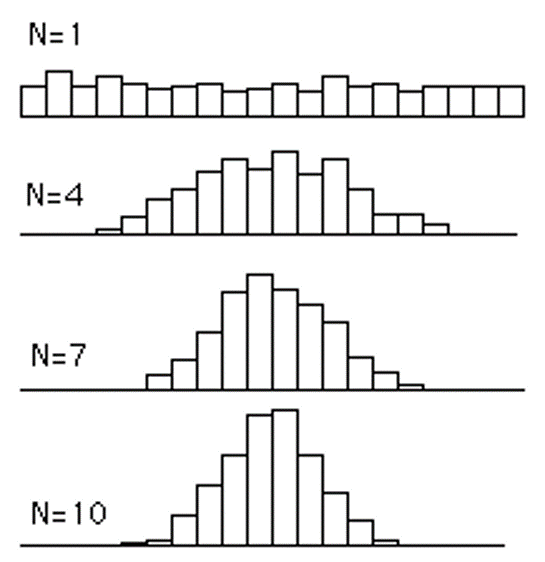Even if the raw data are not normally distributed, the sampling distribution of the mean will tend to be so. This tendency increases as the size of the samples used to generate the means increases.

7

95% confidence interval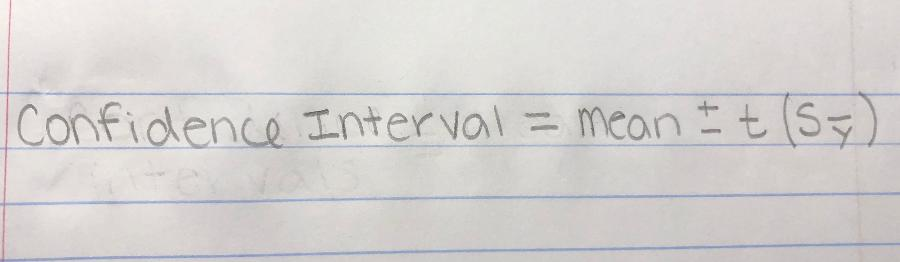Has a very good chance of including the population mean between its upper and lower confidence limits.

8

Upper confidence limit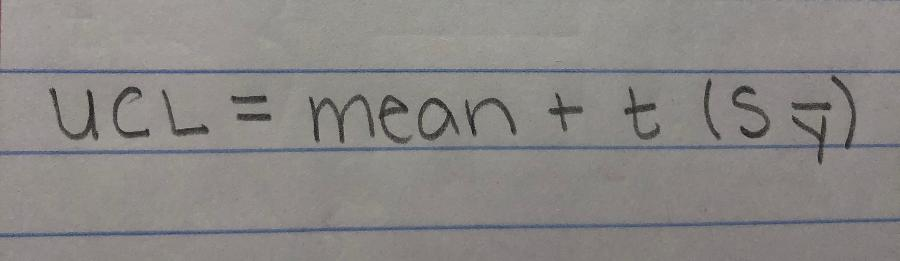9

Lower confidence limit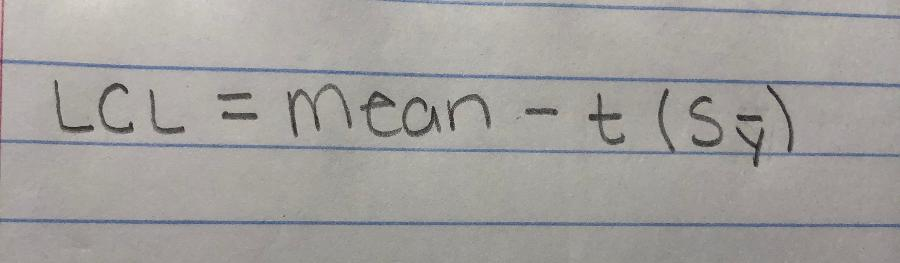10

How do you calculate the value of t?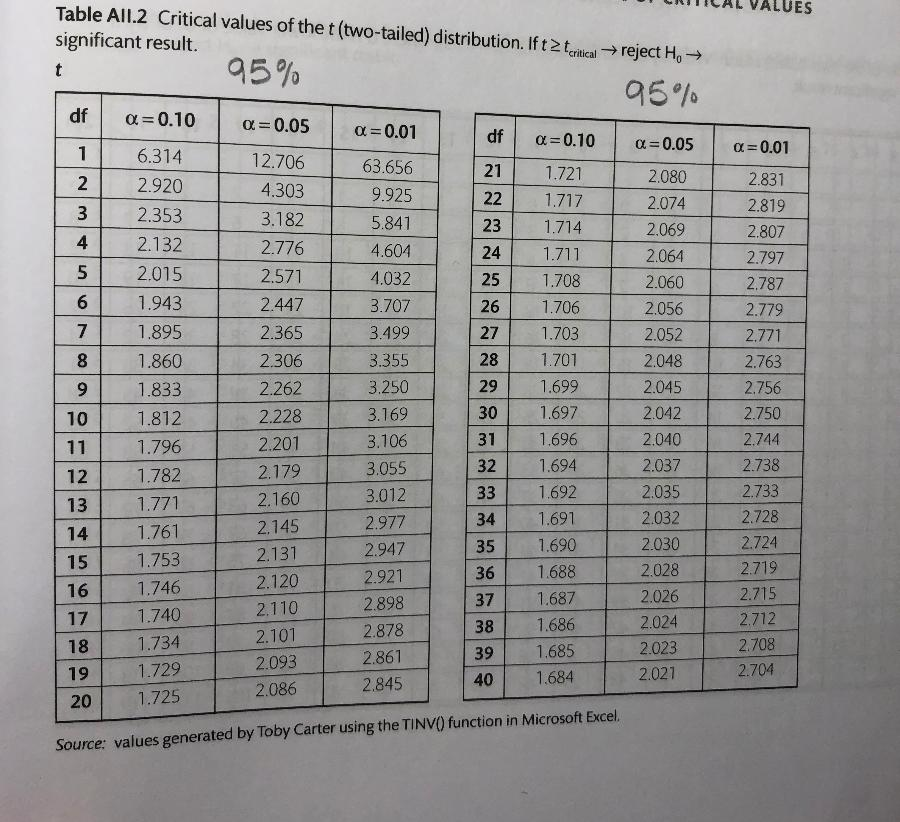Using a critical t value table.

Use the degrees of freedoms of your data and 0.05

11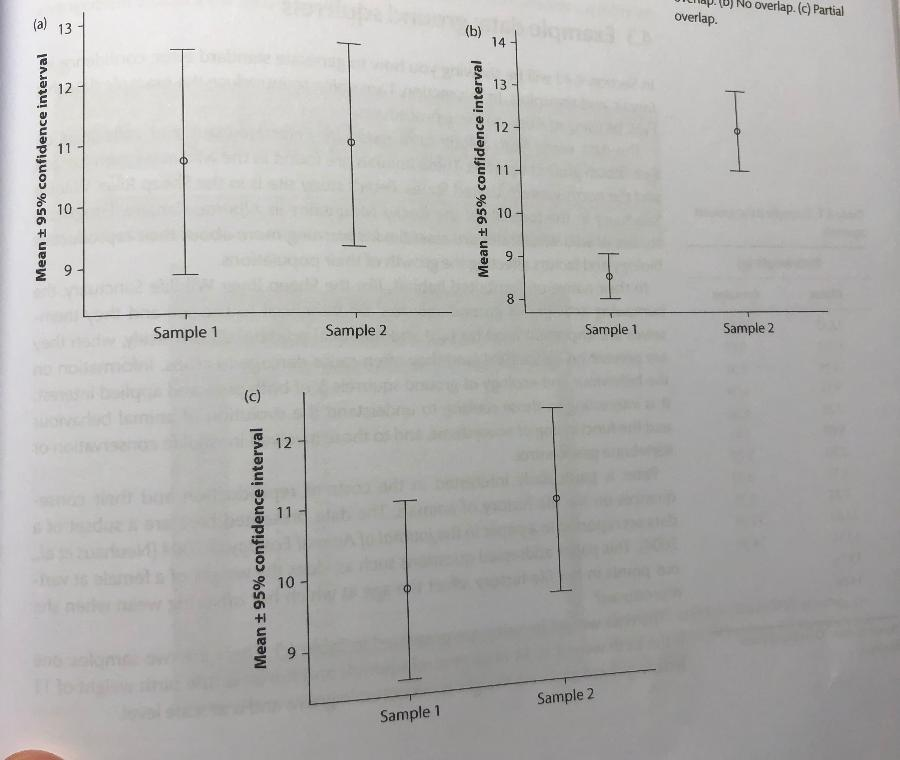Three plots showing the 95% confidence intervals of the mean of samples.

What can you tell from each graph?Graph A - Complete overlap of the bars implies it is very likely that the two samples came from the same population and any difference between them is due to error alone.

Graph B - No overlap of the bars implies it is very unlikely that the samples came from the same population and the difference between them is statistically significant.

Graph C - Intermediate overlap implies that the samples might or might not have come from the same population